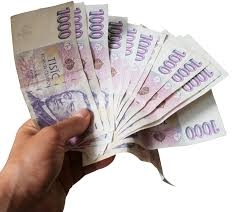# Savings 31311

Petr spent a fifth of his savings on a compact disc and 25% on a book on nature. He has 550 CZK left. How many crowns did Petr save?

x =  1000

### Step-by-step explanation:

x-x/5-0.25x = 550

x-x/5-0.25·x = 550

2.75x = 2750

x = 2750/2.75 = 1000

x = 1000

Our simple equation calculator calculates it.Did you find an error or inaccuracy? Feel free to write us. Thank you!

Tips for related online calculators
Need help calculating sum, simplifying, or multiplying fractions? Try our fraction calculator.